# CompareTransformers

Transformer circuit to show the magnetization facilities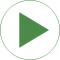# Information

This information is part of the Modelica Standard Library maintained by the Modelica Association.

This example is to demonstrate the behaviour of transformer models. The Basic.Transformer, which consists of mutual coupled inductors, is compared with the ideal transformer model. If the ideal model is used with considerMagnetization=true leakage inductances are taken into account, otherwise not. The example is constructed in such a way that the ideal transformer circuit with considerMagnetization=true shows the same behaviour as the basic transformer.

Simulate until T=50 s with both considerMagnetization=false and considerMagnetization=true of the ideal transformer. Plot in separate windows for comparison:
basicTransformer.p1.v and idealTransformer.p1.v
basicTransformer.p1.i and idealTransformer.p1.i
basicTransformer.p2.v and idealTransformer.p2.v basicTransformer.p2.i and idealTransformer.p2.i

# Parameters (14)

Vdc Value: 0.1 Type: Voltage (V) Description: DC offset of voltage source Value: 0.1 Type: Voltage (V) Description: Peak voltage of voltage source Value: 10 Type: Frequency (Hz) Description: Frequency of voltage source Value: pi / 2 Type: Angle (rad) Description: Phase of voltage source Value: 2 Type: Real Description: Turns ratio primary:secondary voltage Value: 0.01 Type: Resistance (Ω) Description: Primary resistance w.r.t. primary side Value: 0.05 / (2 * pi * f) Type: Inductance (H) Description: Primary leakage inductance w.r.t. primary side Value: 10. / (2 * pi * f) Type: Inductance (H) Description: Magnetizing inductance w.r.t. primary side Value: 0.05 / (2 * pi * f) / n ^ 2 Type: Inductance (H) Description: Secondary leakage inductance w.r.t. secondary side Value: 0.01 / n ^ 2 Type: Resistance (Ω) Description: Secondary resistance w.r.t. secondary side Value: 1 / n ^ 2 Type: Resistance (Ω) Description: Load resistance Value: L1sigma + M * n Type: Inductance (H) Description: Primary no-load inductance Value: L2sigma + M / n Type: Inductance (H) Description: Secondary no-load inductance Value: Lm1 / n Type: Inductance (H) Description: Mutual inductance

# Outputs (8)

v1B Default Value: resistor11.n.v Type: Voltage (V) Description: Primary voltage, basic transformer Default Value: resistor11.i Type: Current (A) Description: Primary current, basic transformer Default Value: resistor12.p.v Type: Voltage (V) Description: Secondary voltage, basic transformer Default Value: resistor12.i Type: Current (A) Description: Secondary current, basic transformer Default Value: resistor21.n.v Type: Voltage (V) Description: Primary voltage, basic transformer Default Value: resistor21.i Type: Current (A) Description: Primary current, basic transformer Default Value: resistor22.p.v Type: Voltage (V) Description: Secondary voltage, basic transformer Default Value: resistor22.i Type: Current (A) Description: Secondary current, basic transformer

# Components (16)

sineVoltage1 ground11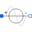Type: SineVoltage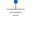Type: Ground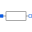Type: ResistorType: ResistorType: ResistorType: GroundType: SineVoltageType: GroundType: Resistor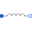Type: InductorType: InductorType: ResistorType: ResistorType: Ground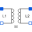Type: Transformer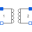Type: IdealTransformer